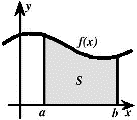## Math in the Middle Institute Partnership## MAT Exam Expository Papers

#### Date of this Version

7-2007

In partial fulfillment of the requirements for the Master of Arts in Teaching with a Specialization in the Teaching of Middle Level Mathematics in the Department of Mathematics. Jim Lewis, Advisor July 2007

#### Abstract

This paper looks at comparing the perimeter and area of inscribed and circumscribed regular polygons. All constructions will be made with circles of radius equal to 1 unit. To begin this exploration, I created a circle with a radius of 1(for my purposes I used 1 inch as my unit of measure). I chose my first construction to contain the most basic regular polygon, an equilateral triangle. A regular polygon implies that all sides of the figure are equal and all interior angles of the figure are congruent. My first construction shows an equilateral triangle inscribed in a circle (see Appendix A). Next, I needed to find the perimeter of this inscribed triangle. I used a ruler to measure the distance of one of the sides, then multiplied that value times 3, the number of sides of the triangle. This method will work for finding the perimeter of all regular polygons. The only change would be substituting three with the number of sides of the polygon being studied. A general formula would be: Perimeter of a regular polygon with n sides equals the length of one side times n.

COinS